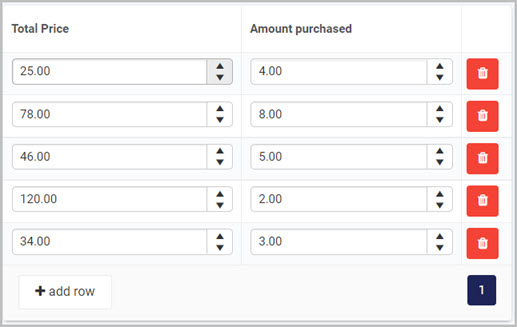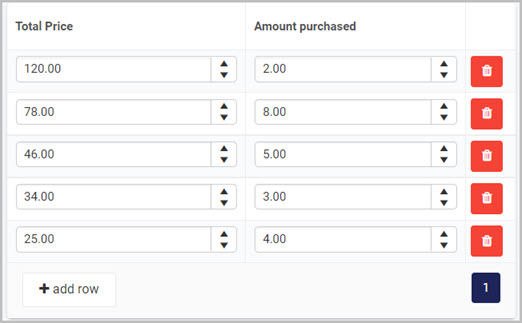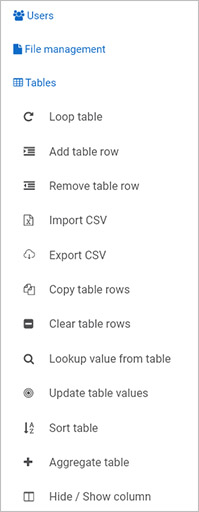# Table rules

Table rules is one category of rules to enable table operations such as sorting, copying table rows to another table, exporting and importing table data as .csv files and adding and removing table rows. These operations are useful to implement in external data sources, automating actions using Kianda processes.

Take an example of a Sort table rule. Implementing this rule will result in a table being sorted in an ascending or descending manner depending on what column of the table you want to sort by. For an example, take the image below as your table.You can attach the Sort table rule to a button and selecting one of the columns available, for example Total Price, and the order of sorting for example descending. The result of the table after sorting will look as follows:## Getting started with Table rules

If you go to Administration > Designer and click on a process or create a new process, then click on Add a rule, the Table rules are found in the left-hand pane when you click on Tables.There are 12 types of Table rules as follows:

• Loop table - this rule loops through table rows, where other rules can be triggered and implemented.

• Add table row - this rule adds a new row to a table.

• Remove table row - this rule removes a current row from a table.

• Import CSV - this rule imports .csv or .xlsx file data into a table, based on mapping provided within the rule.

• Export CSV - this rule is export the contents of a table to a .csv file.

• Copy table rows - this rule copies table rows from one table to another.

• Clear table rows - this rule removes table rows that match given criteria.

• Lookup value from table - this rule performs a lookup on a table value that match given criteria

• Update table values -this rule updates table columns that match given criteria.

• Sort table -this rule sorts table data based on multiple conditions.

• Aggregate table - this rule aggregates table values.

• Hide/Show column - this rule allows you to hide or show columns within a table